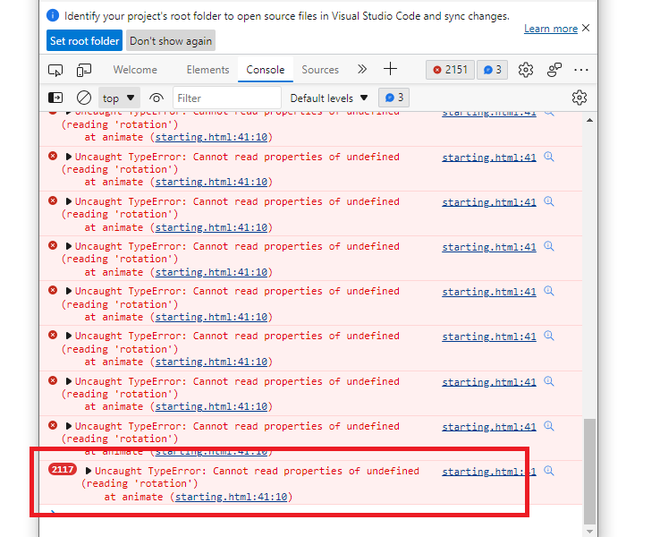Open in App
Not now

# Object is undefined” error when trying to rotate object in three.js

• Last Updated : 07 Nov, 2022

In this article, we will see the “Uncaught TypeError: Cannot read properties of undefined (reading ‘rotation’) at animate”. It is a type error for a variable, in Three .js, this error occurs when the variable is declared, but the value is not assigned or the value is undefined. We can observe from the error, message that this error occurred, when the variable was invoked, for amending the rotation, to animate

The variable here is an instance of THREE.Object3D

Example: This example, creates the above-shown error i.e. “Uncaught TypeError: Cannot read properties of undefined (reading ‘rotation’)at animate”.

This is a three.js code that creates, a cone, with rotation in the x and y-axis. Here, the variable name ‘cone’ has been, declared, but not assigned with a 3D object. Because of this when we try to access, the rotation key of the variable, it is not able to read it, hence, shows an error.

## HTML

 ```<``html``>`` ` `<``head``>``    ``<``meta` `charset``=``"utf-8"``>``    ``<``title``>``        ``Solving errors with``        ``geeksforgeeks in three.js``    ```` ` `    ``<``script` `src``=``"three.js"``>```` ` `<``body``>``    ``<``script``>`` ` `        ``// Adding scene and camera ``        ``const scene = new THREE.Scene();``        ``const camera = new THREE.PerspectiveCamera(``            ``75, window.innerWidth / window.innerHeight, ``            ``0.1, 1000);`` ` `        ``// Adding rendering ``        ``const renderer = new THREE.WebGLRenderer();``        ``renderer.setSize(window.innerWidth, ``                ``window.innerHeight);``        ``document.body.appendChild(renderer.domElement);`` ` `        ``// Creating a cone geometry, and material. ``        ``const geometry = new THREE.ConeGeometry();``        ``const material = new THREE.MeshBasicMaterial({``            ``color: 'purple'``        ``});`` ` `        ``// Declaring Variable``        ``let cone;`` ` `        ``// Adding cone to the scene. ``        ``scene.add(cone);`` ` `        ``camera.position.z = 7;`` ` `        ``// Writing an animate() function. ``        ``function animate() {``            ``requestAnimationFrame(animate);`` ` `            ``// Cone not assigned, with a ``            ``// 3D object but still trying ``            ``// to rotate an, undefined object.``            ``cone.rotation.x += 0.01;``            ``cone.rotation.y += 0.01;``            ``renderer.render(scene, camera);``        ``};``        ``animate();``    `````` ` ``

Output:To solve this error, we need to create a Mesh, which combines, the geometry and material declared previously. The Mesh function is assigned to the variable ‘cone’, hence the error gets removed. The value assigned is, “new THREE.Mesh( geometry, material )”

Example: The below example, resolves the error, by assigning value to the ‘cone’

## HTML

 ```<``html``>`` ` `<``head``>``    ``<``meta` `charset``=``"utf-8"``>``    ``<``title``>``        ``Solving errors with``        ``geeksforgeeks in three.js``    ```` ` `    ``<``script` `src``=``"three.js"``>```` ` `<``body``>``    ``<``script``>`` ` `        ``// Adding scene and camera ``        ``const scene = new THREE.Scene();``        ``const camera = new THREE.PerspectiveCamera(``            ``75, window.innerWidth / window.innerHeight, ``            ``0.1, 1000);`` ` `        ``// Adding rendering ``        ``const renderer = new THREE.WebGLRenderer();``        ``renderer.setSize(window.innerWidth, window.innerHeight);``        ``document.body.appendChild(renderer.domElement);`` ` `        ``// Creating a cone geometry, and material. ``        ``const geometry = new THREE.ConeGeometry();``        ``const material = new THREE.MeshBasicMaterial({``            ``color: 'purple'``        ``});`` ` `        ``// Declaring variable and assigning its value``        ``const cone = new THREE.Mesh(geometry, material);`` ` `        ``// Adding cone to the scene. ``        ``scene.add(cone);`` ` `        ``camera.position.z = 7;`` ` `        ``// Writing an animate() function. ``        ``function animate() {``            ``requestAnimationFrame(animate);`` ` `            ``// Adding rotations, in x and y direction,``            ``// in the cone.``            ``cone.rotation.x += 0.01;``            ``cone.rotation.y += 0.01;`` ` `            ``renderer.render(scene, camera);``        ``};``        ``animate();``    `````` ` ``

Output:My Personal Notes arrow_drop_up